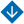Estimating Time Characteristics of Control Systems with Cyclic Operation: A Network Calculus Approach
•About Advisory & Editorial Boards Aims & Scope Publication Ethics Review Process Archive Contact Information

#Estimating Time Characteristics of Control Systems with Cyclic Operation: A Network Calculus Approach

Promyslov, V.G. and Semenkov, K.V. Estimating Time Characteristics of Control Systems with Cyclic Operation: A Network Calculus Approach
Abstract. The practical validation of time characteristics of digital control systems is considered. The delay in information processing and transmission often has a probability distribution differing from the Gaussian one. Therefore, the confidence intervals calculated under the Gaussian distribution assumption will be incorrect for such systems. The idea is to estimate the time characteristics of a control system using non-statistical time parameter estimation methods. As one of such methods, Network Calculus is considered. The practical implementation of Network Calculus to estimate the parameters of control systems, particularly its features, is discussed. One of the main features is imposing special restrictions on data flows and system performance, determined by flow envelopes and maximum (minimum) service curves. Generally, these characteristics are unknown in advance. Mathematical methods are proposed to estimate these characteristics under known input and output data flows in the system. As shown below, the calculation of characteristics is significantly simplified for systems with cyclic data processing algorithms, and the data transfer rate over the network is much higher than that on the computing components of the system. Simulations are carried out, and the system’s time parameters estimated by Network Calculus are compared with the results of classical statistical estimation methods. As an illustrative example, the time parameters of one component of a real nuclear power plant instrumentation and control system are estimated using Network Calculus.

Keywords: system performance, time characteristics, digital control systems, Network Calculus, non-statistical estimation methods.

Funding. This work was supported in part by the Russian Foundation for Basic Research, project no. 19-29-06044 (Sections 2 and 3).

PDF (English)